# Unitary Matrix Networks in the Time Domain¶

## Imports¶

:

# Photontorch
import photontorch as pt

# Python
import torch
import numpy as np
import matplotlib.pyplot as plt
from tqdm.notebook import trange

# numpy settings
np.random.seed(6) # seed for random numbers
np.set_printoptions(precision=2, suppress=True) # show less numbers while printing numpy arrays


## Schematic¶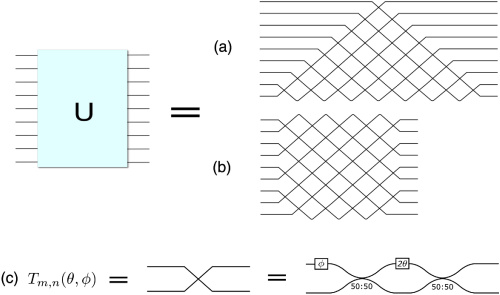1. Reck Design

2. Clements Design

## Simulation and Design Parameters¶

Here we will use the matrix network with delays.

:

N = 4
length = 25e-6 #[m]
transmission = 0.5 #[]
neff = 2.86
env = pt.Environment(
t_start = 0,
t_end = 2000e-14,
dt = 1e-13,
wl = 1.55e-6,
)
pt.set_environment(env)

source = torch.ones(N, names=["s"])/np.sqrt(N) # Source tensors with less than 4D need to have named dimensions.

env

:

key value description
nameenvname of the environment
t[0.000e+00, 1.000e-13, ..., 1.980e-11][s] full 1D time array.
t00.000e+00[s] starting time of the simulation.
t11.990e-11[s] ending time of the simulation.
num_t199number of timesteps in the simulation.
dt1.000e-13[s] timestep of the simulation
samplerate1.000e+13[1/s] samplerate of the simulation.
bitrateNone[1/s] bitrate of the signal.
bitlengthNone[s] bitlength of the signal.
wl1.550e-06[m] full 1D wavelength array.
wl01.550e-06[m] start of wavelength range.
wl1None[m] end of wavelength range.
num_wl1number of independent wavelengths in the simulation
dwlNone[m] wavelength step sizebetween wl0 and wl1.
f1.934e+14[1/s] full 1D frequency array.
f01.934e+14[1/s] start of frequency range.
f1None[1/s] end of frequency range.
num_f1number of independent frequencies in the simulation
dfNone[1/s] frequency step between f0 and f1.
c2.998e+08[m/s] speed of light used during simulations.
freqdomainFalseonly do frequency domain calculations.

## A. Reck Design¶

:

nw = pt.ReckNxN(
N=N,
wg_factory=lambda: pt.Waveguide(length=1e-4, phase=2*np.pi*np.random.rand(), trainable=True),
mzi_factory=lambda: pt.Mzi(length=1e-4, phi=2*np.pi*np.random.rand(), theta=2*np.pi*np.random.rand(), trainable=True),
).terminate()


## Simulation¶

:

detected_time = nw(source)
nw.plot(detected_time[:,0,:,0]); # plot first and only batch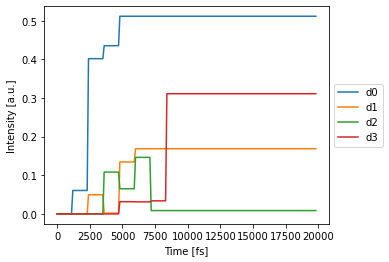## Total power recovered:¶

:

detected_time[-1,0,:,0].sum()

:

tensor(1.0000)


## Optimizing the coupling¶

The goal is to optimize the coupling of the network such that we have the same output at the 4 detectors with an as high as possible amplitude (ideally, higher than in the equal coupling case).

:

def train_for_same_output(nw, num_epochs=50, learning_rate=0.1):
target = torch.tensor([1.0/N]*N, device=nw.device)
lossfunc = torch.nn.MSELoss()
optimizer = torch.optim.Adam(nw.parameters(), lr=learning_rate)
with pt.Environment(wl=1.55e-6, t0=0, t1=10e-12, dt=1e-13, grad=True):
range_ = trange(num_epochs)
for epoch in range_:
det_train = nw(source)[-1,0,:,0]
loss = lossfunc(det_train, target)
loss.backward()
optimizer.step()
range_.set_postfix(loss=loss.item())

train_for_same_output(nw)




## Final Simulation¶

:

%time det_train = nw(source)
nw.plot(det_train[:,0,:,0]); # plot first and only batch

CPU times: user 42.5 ms, sys: 77 µs, total: 42.6 ms
Wall time: 41.9 ms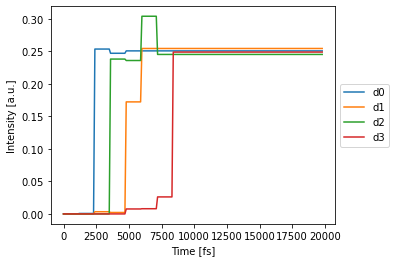Note that in the Reck network, signals arrive at different times.

## Total power recovered¶

:

det_train[-1,0,:,0].sum()

:

tensor(1.)


## B. Clements Design¶

:

nw = pt.ClementsNxN(
N=N,
capacity=N,
wg_factory=lambda: pt.Waveguide(length=1e-4, phase=2*np.pi*np.random.rand(), trainable=True),
mzi_factory=lambda: pt.Mzi(length=1e-4, phi=2*np.pi*np.random.rand(), theta=2*np.pi*np.random.rand(), trainable=True),
).terminate()


## Simulation¶

:

detected_time = nw(source)
nw.plot(detected_time[:,0,:,0]); # plot first and only batch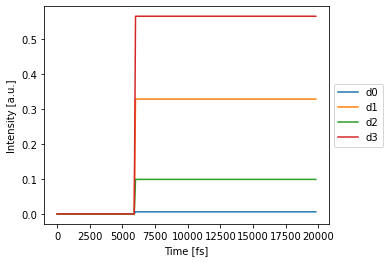## Total power recovered:¶

:

detected_time[-1,0,:,0].sum()

:

tensor(1.)


## Optimizing the coupling¶

:

def train_for_same_output(nw, num_epochs=50, learning_rate=0.1):
target = torch.tensor([1.0/N]*N, device=nw.device)
lossfunc = torch.nn.MSELoss()
optimizer = torch.optim.Adam(nw.parameters(), lr=learning_rate)
with pt.Environment(wl=1.55e-6, t0=0, t1=10e-12, dt=1e-13, grad=True):
range_ = trange(num_epochs)
for epoch in range_:
det_train = nw(source)[-1,0,:,0] # get first and only batch
loss = lossfunc(det_train, target)
loss.backward()
optimizer.step()
range_.set_postfix(loss=loss.item())

train_for_same_output(nw)




## Final Simulation¶

:

%time det_train = nw(source)
nw.plot(det_train[:,0,:,0]); # plot first and only batch

CPU times: user 41.1 ms, sys: 21 µs, total: 41.1 ms
Wall time: 40.4 ms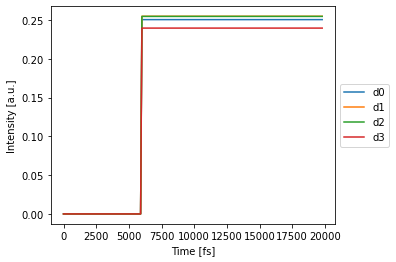Note that in the Clements network, all signals arrive at the same time at the detector.